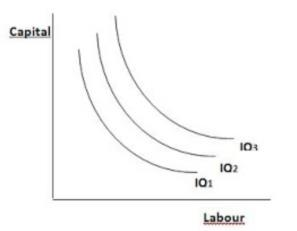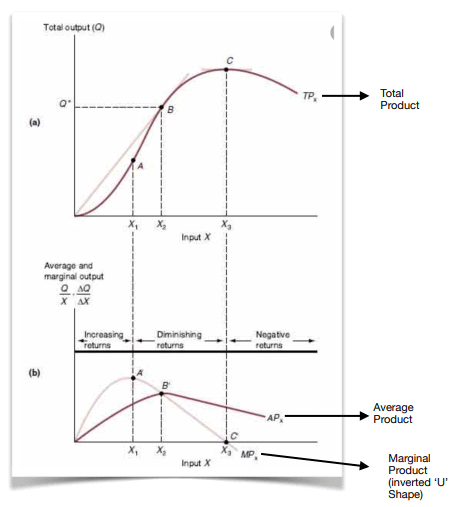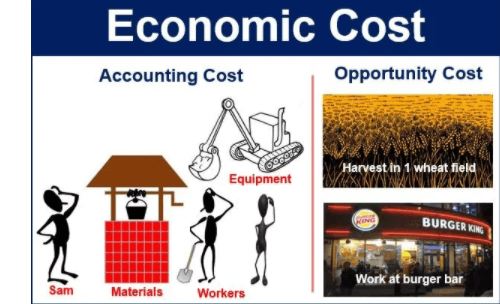Courses

# Basics of Microeconomics- 2 UPSC Notes | EduRev

## UPSC : Basics of Microeconomics- 2 UPSC Notes | EduRev

The document Basics of Microeconomics- 2 UPSC Notes | EduRev is a part of the UPSC Course Indian Economy for UPSC CSE.
All you need of UPSC at this link: UPSC

Production Function/Supply Side

• In economics, a production function gives the technological relation between quantities of physical inputs and quantities of output of goods.
• So, let’s suppose there are two inputs used by a producer to produce a given quantity of goods.
• Inputs = Labour (L) & Capital(K)
• Output is denoted by Q
• So a production function would look like this: Q = f (L,K)

Generally, each production unit uses 4 types of inputs, which are also known as Factors of Production:

• Land
• Labour
• Capital
• Entrepreneurship

A producer does not get these Factors of Production for free, they come at a price:

• For Land he pays Rent
• For Labour he pays wages
• For Capital, interest is paid
• For Entrepreneurship, proﬁt is paid.

Rent, Wages, Interest and Proﬁt are therefore known as Factor Prices or Factor Incomes

While talking about the above mentioned Production Function, we have taken following assumptions:

• Eﬃcient use of all inputs [inputs are utilised at their full eﬃciency], and
• A Production Function is deﬁned for a given technological level.

What is an Isoquant?

• An Isoquant is a set of all possible combinations of two inputs [labour & capital] that yield the same level of output.
• The Isoquant curve is a graph, used in the study of Microeconomics, that charts all inputs that produce a speciﬁc level of output.
• So, one Isoquant represents all possible combinations of labour and capital, that will yield similar output.
• This graph is used as a metric for the inﬂuence that the inputs have on the level of output or production that can be obtained.
•  The Isoquant curve assists ﬁrms in making adjustments to inputs to maximize outputs, and thus proﬁts.Some important properties of Isoquant curve are as following:

• The curve is generally downward or negative sloping
• The curve is generally convex to the origin
• Isoquant curves cannot intersect or be tangent to one another
• The higher or outer the curve, the higher is the output. For example, in the above diagram, IQ3 has the highest output, then IQ2 and IQ1 has the lowest output.
• Isoquant curves cannot touch either of the axes (X or Y)

Total, Average and Marginal Product:

• As we all know that in a production process we need a particular proportion of inputs to get a speciﬁc output.
• For a ﬁrm, these inputs are the factors of production [land, labour, capital and entrepreneurship].
• To know the contribution of a particular input, we keep all the other inputs constant and vary that particular input to reveal the relation of that input with the output.
• So, if we want to know the relation of labour with the total output of a ﬁrm, we make use of Total, Average and Marginal Product of Labour
• Total Product = Total return on a variable input
• Average Product = Output per unit variable input [ Total Product / Total units of variable input]
• Marginal Product = It is deﬁned as the change in output per unit change in variable input [change in total product / change in variable input].• Law of Diminishing Marginal Product - It says that if we keep increasing the employment of an input, with other inputs ﬁxed, eventually after a point, Marginal Product of that input will start to decline.
• Law of Variable Proportions - This law states that if we keep one factor input variable and others constant, the marginal product curve of that input will follow an inverted ‘U’ shape.

In the above section we discussed the impact on output by changes in the variable input. Now, in this section we are going to talk about impact on output when all inputs are kept variable and none is ﬁxed.
On the basis of changes in all the inputs, a Production Function can be classiﬁed in 3 ways:

• Constant Return to Scale: A production function under which, a proportional increase in all inputs results in an increase in the output by the same proportion.
Example - Eg - 2Q = f (2L, 2K)
• Increased Return to Scale: A production function under which, a proportional increase in all the inputs results in an increase in output by a proportion greater than that with which the inputs were increased.
Example - 10Q = f (8L, 8K)
• Decreased Return to Scale: A production function under which, a proportional increase in all the inputs results in an increase in output by a proportion less than that with which the inputs were increased.
Example - 3Q = F(8L, 8K).

Concept of Cost of Production:

• Average Cost of Production = Total cost of production / Total Number of units produced
• Marginal Cost of Production = Change in Total Cost / Change in total units produced
• Fixed Cost / Overhead Cost - These are costs which are incurred in hiring ﬁxed factors of production whose amount cannot be altered in the short run. For example costs incurred in setting up of production plant or cost of buying machinery.
• Variable Cost / Prime Cost / Direct Cost - These are costs incurred on employment of variable factors of production whose amount can be altered in the short run. For example, costs incurred on labour, raw material etc.
• Total Cost = Total Fixed Cost + Total Variable Cost

Opportunity, Accounting and Economic Cost:

• Opportunity cost - Opportunity or Alternative cost of making a particular choice is the value of the most valuable choice out of those that were not taken. It is the cost incurred by not enjoying the beneﬁt associated with the next best alternative choice.
• Accounting Cost - These are the explicit costs incurred in a choice that one has chosen.
• Economic Cost = Opportunity Cost + Accounting CostEconomic Cost = 20 + 10 = 30

Market Structure

The structure of markets can be basically diﬀerentiated on the basis of two major features

• Number of buyers and sellers in the market
• Is the product being sold in the market Homogeneous or Diﬀerentiated?

Homogeneous Products - It is a product that cannot be distinguished from competing products from diﬀerent suppliers. In other words, the product has essentially same physical characteristics and quality as similar products from other suppliers. One product can be easily substituted for the other.
Diﬀerentiated Product - Product diﬀerentiation is the process of distinguishing a product or a service from other computing products or services. Thus, diﬀerentiated products are those that can be distinguished from competing products by other suppliers.
Example - Taj tea and TATA tea.

Diﬀerent Types of Market Structures are as following
1. Perfect Competition

• Under this form of market structure, there are a large number of producers as well as buyers.
• The products that are being sold in such a market are homogeneous.
• There are no entry or exit barriers for ﬁrms. That means free entry and exit for ﬁrms.
• The demand in such a market is ‘perfectly elastic’ i.e. inﬁnitely elastic.
• Both consumers and producers are price takers, and the producers have zero control over price and quantity of production.

2. Monopolistic Competition

• Large number of buyers and sellers
• Unlike perfect competition, the products here are diﬀerentiated.
• Because of Product Diﬀerentiation, the producers have some form of monopoly and control over price of their product [though very little control, but more than perfect competition].
• All products by diﬀerent sellers in the market are close substitutes of each other.

3. Oligopoly

• Under this form of market structure, there are very few number of big producers who control the market.
• Each producer has a large market share.
• On the basis of product diﬀerentiation, Oligopoly can be further subdivided into 2 parts:
Pure Oligopoly = When products are homogeneous
Diﬀerentiated Oligopoly = When products are diﬀerentiated

4. Monopoly

• One producer with complete control over market share.
• Single unique product with no close substitutes.
• This market structure is the complete polar opposite of perfect competition.
• Here, the producer has complete control over price and production levels.

Concept of Economy of Scale

It is the cost advantage that enterprises obtain due to their scale of operation, with cost per unit of output declining with increasing scale of production.

Therefore, the concept of economy of scale states that as a production unit gets bigger, its average cost comes down and that unit becomes more eﬃcient.

Offer running on EduRev: Apply code STAYHOME200 to get INR 200 off on our premium plan EduRev Infinity!

## Indian Economy for UPSC CSE

109 videos|196 docs|84 tests

,

,

,

,

,

,

,

,

,

,

,

,

,

,

,

,

,

,

,

,

,

;